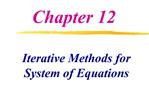DownloadDownload PresentationIterative Methods for System of Equations

# Iterative Methods for System of Equations

Télécharger la présentation## Iterative Methods for System of Equations

- - - - - - - - - - - - - - - - - - - - - - - - - - - E N D - - - - - - - - - - - - - - - - - - - - - - - - - - -
##### Presentation Transcript

1. Chapter 12 Iterative Methods for System of Equations

2. Iterative Methods for Solving Matrix Equations Jacobi method Gauss-Seidel Method* Successive Over Relaxation (SOR) MATLAB�s Methods

3. Iterative Methods

4. Idea behind iterative methods: Convert Ax = b into x = Cx +d Generate a sequence of approximations (iteration) x1, x2, �., with initial x0 Similar to fix-point iteration method Iterative Methods

5. Rearrange Matrix Equations Rewrite the matrix equation in the same way

6. Iterative Methods x and d are column vectors, and C is a square matrix

7. Convergence Criterion For system of equations

8. Jacobi Method

9. Gauss-Seidel Method

10. Gauss-Seidel Method

11. (a) Gauss-Seidel Method (b) Jacobi Method

12. Convergence and Diagonal Dominant Sufficient condition -- A is diagonally dominant Diagonally dominant: the magnitude of the diagonal element is larger than the sum of absolute value of the other elements in the row Necessary and sufficient condition -- the magnitude of the largest eigenvalue of C (not A) is less than 1 Fortunately, many engineering problems of practical importance satisfy this requirement Use partial pivoting to rearrange equations!

14. Diagonally Dominant Matrix

15. Jacobi and Gauss-Seidel

16. Example

17. Gauss-Seidel Iteration

18. Gauss-Seidel Method

19. MATLAB M-File for Gauss-Seidel method

20. MATLAB M-File for Gauss-Seidel method

23. Relaxation Method Relaxation (weighting) factor ? Gauss-Seidel method: ? = 1 Overrelaxation 1 < ? < 2 Underrelaxation 0 < ? < 1 Successive Over-relaxation (SOR)

24. Successive Over Relaxation (SOR) Relaxation method

25. SOR Iterations

26. SOR Iterations Converges slower !! (see MATLAB solutions) There is an optimal relaxation parameter

30. CVEN 302-501 Homework No. 8 Chapter 12 Prob. 12.4 & 12.5 (Hand calculation and check the results using the programs) You do it but do not hand in. The solution will be posted on the net.

31. Nonlinear Systems Simultaneous nonlinear equations Example

32. Two Nonlinear Functions

34. Newton-Raphson Method One nonlinear equation (Ch.6) Two nonlinear equations (Taylor-series)

35. Intersection of Two Curves Two roots: f1(x1,x2) = 0 , f2 (x1,x2) = 0 Alternatively

36. Intersection of Two Curves Intersection of a circle and a parabola

37. Intersection of Two Curves

42. Newton-Raphson Method n nonlinear equations in n unknowns

43. Jacobian (matrix of partial derivatives) Newton�s iteration (without inversion) Newton-Raphson Method

44. For a single equation with one variable Newton�s iteration Newton-Raphson Method

47. Intersection of Three Surfaces Solve the nonlinear system Jacobian

48. Newton-Raphson Method Solve the nonlinear system MATLAB function ( y = J-1F )

50. No need to compute partial derivatives Fixed-Point Iteration

51. Example1: Fixed-Point Solve the nonlinear system Rearrange (initial guess: x = y = z = 2)

54. Example 2: Fixed-Point Solve the nonlinear system Rearrange (initial guess: x = 0, y = 0, z > 0)